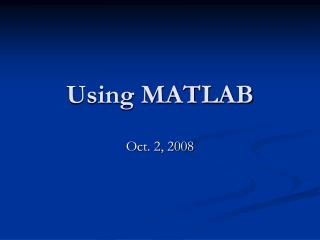DownloadDownload PresentationUsing MATLAB

# Using MATLAB

Download Presentation## Using MATLAB

- - - - - - - - - - - - - - - - - - - - - - - - - - - E N D - - - - - - - - - - - - - - - - - - - - - - - - - - -
##### Presentation Transcript

1. Using MATLAB Oct. 2, 2008

2. About Matlab • Origins are in linear algebra • Much functionality added later • Runs on all platforms • Many toolboxes exist

3. The User Interface • You can use Matlab interactively • Just type commands and view results • Difficulty is saving session • To use scripts (m-files) • To use the built-in editor

4. The Approach • Put commands into m-file • Run from main Matlab window • Edit m-file • Rerun • Repeat to perfection • Save and turn in m-file

5. Variables • A=5 • B=3 • C=A+B • C=C+3

6. Vectors and Matrices • Think of vectors as lists • Think of matrices as arrays (lists of lists) • V1=[0 1 2 3 4] • V2=0:4 • M1=[1 0 1; 0 1 0; 0 0 1] • M2=ones(3)

7. Accessing elements • You can pick out individual components of vectors and matrices • V1(3) • M1(2,3) • M1(:,2) • M1(1,:)

8. Vector Math • Try this: • V=0:5 • Z=V*V • To square each element: • Z=V.*V • Also ./ and .^

9. Plotting • Make vectors for x and y axis and the plot them x=0:0.1:10 y=sin(x) plot(x,y) plot(x,y,x,y,’o’)

10. Functions • exp, log, log10, sqrt • sin, cos, tan, asin, acos, atan • max, min, mean, median, sum, prod, sort

11. Flow Control if x<10 then x=x+1 else x=x^2 end

12. Flow Control (cont) for i=1:10 z=z*I end

13. User-Defined Functions • Suppose we want to plot: sin(3*x)+sin(3.1*x) • Create user-defined function function r=f(x) r= sin(3*x)+sin(3.1*x) • Save as f.m

14. Conclusions • This should get you started with Matlab • help help+command